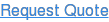An inductor is an electromagnetic component used to resist the changes in current in a circuit. It consists of a wire wound into a coil, which temporarily stores energy in a magnetic field — they are like an electrical version of a flywheel. When the current in the circuit changes, the magnetic field induces a voltage in the conductor in the opposite direction of the incoming current. In other words, inductors oppose changes in current.When the magnetic field is fully built up, current can flow normally — and uniformly — through the conductor. When the circuit is opened, current will continue to flow until the inductor is drained of its magnetic field.

## Basic Theory

Inductors are rated based on their inductance — the ratio of the voltage to the rate of current change, measured in Henries (H). When current passes through an inductor, a magnetic flux is created. Inductance is determined by the ratio of magnetic flux (in Gauss of Tesla) to the current entering the inductor (in amps). For inductors with ferromagnetic cores, the inductance changes nonlinearly. In this case, the inductance is the ratio of the derivative (rate of change) of the magnetic flux to the derivative of the current.

Basically, the inductance depends on the shape of the current path. Inductors are generally shaped as a coil or a helix — the more turns there are around the core, the greater the inductance. Inductance is also dependent other factors, such as the length of the coil, the area of the coil, the space between each coil turn, and most importantly, the core material.

## Basic Construction

Inductors consist of a magnetic iron or ferrite core inside an insulated copper coil. For high frequency applications, ferrite or powdered (distributed gap) cores are used. The high permeability of the ferromagnetic core creates a large magnetic field and, therefore, a greater level of inductance.

Low frequency inductors can be built more in the style of transformers, featuring cores of electrical steel.

## Different Types & Applications of Inductors

There are several different types of inductors available, common mode and differential mode filters, power factor correction, LLC, and more. Each type uses the same basic principles, but vary in their materials and particular applications.

Inductors are commonly used in analog circuits, signal processing, and AC electronic equipment. Large inductors are used in power transmission systems to remove current output fluctuations. Smaller inductors are used to prevent radio frequency interference in electronic circuits, or to smooth out low frequency voltages in DC power supplies.

Lastly, when inductors are combined with capacitors, they create a “tuned” circuit, acting as a resonator for oscillating currents. Tuned circuits are commonly used in radio transmitters and receivers.